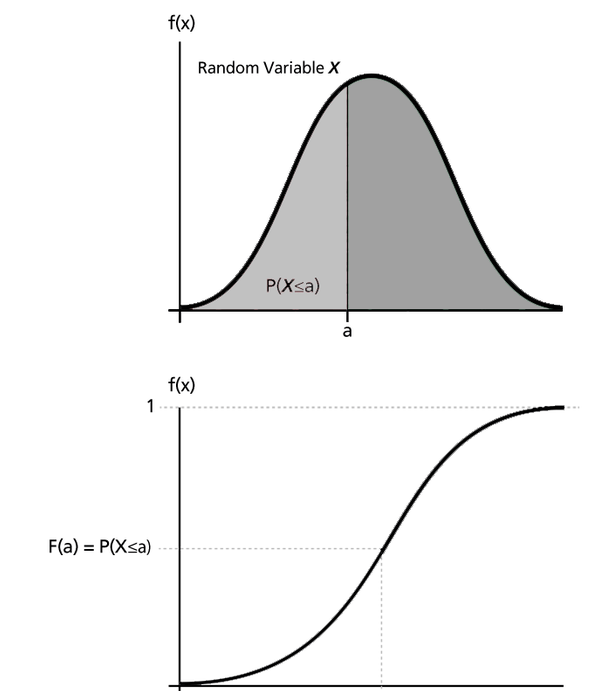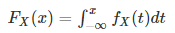##### Introduction

Statistical inference is heavily reliant on the Probability Density Function(PDF), as well as its function brother, the Cumulative Distribution Function(CDF). Let us first understand more about PDF.

What Is A Probability Density Function?

A CDF is often much more complicated than a PDF. A CDF is usually associated with PDF-distributed data. But what is PDF-distributed data? Basically, it’s data that has been distributed using a function. Such functions are used in statistics to determine certain attributes of the data. Once those attributes are determined, a formula is used to determine the overall feature. Data distribution is then determined. The PDF of the normal distribution can be computed by subtracting mu (the mean) from xbar (the current sample) divided by sigma (the standard deviation).

##### Explain Cumulative Distribution Function

The Cumulative Distribution Function (CDF), of a real-valued random variable X, evaluated at x, is the probability function that X will take a value less than or equal to x. Tables can be used to describe the probability distribution of random variables. A CDF plot can easily be made in an Excel worksheet using these data. The CDF method calculates the probability of a given value based on the cumulative distribution function. Under certain conditions, it is used to determine the probability of a random variable and to compare the probability of different values. When dealing with discrete distribution functions, CDF provides the probability values up to the specified number, and when dealing with continuous distribution functions, it provides the area under the probability density function up to the given value.

Distribution functions of variables are essentially just representations of the probability that a variable will take a value an equal or less than a given value. Such a value, of course, is derived from continuous statistical features. What makes a CDF unique is its monotonicity. In particular, monotonic increasing. As time goes on, this probability will always rise.

Therefore, CDFs are typically used to render a continuous distribution as a scalar. Continuous probability distributions (CPDs) have uncountable elements. Each has its own cumulative distribution function, which means they are unique.

Based on this, the probability for each subset of support can be calculated. Probably one of the best known examples of this type of distribution is the normal distribution. Uniform distributions are also continuous probability distributions. Mathematically, this means

Fx(x) = P(X <= x)

X takes the value less than or equal to X if the right side of the equation is less than or equal to X. This is the probability that X lies between (a, b) where a < b. The probability that X is less than or equal to x is what we are checking. The line will continue to go straight if this is true. Otherwise, we will ascend. The hill-like shape of the CDF is precisely a result of this process. This is also the reason why it is monotonic non-decreasing. Additionally, CDFs always have right-continuous behaviour.

In other words, the values are continuous from left to right. We could plot them as progressive increases from left to right if we were to plot them.

The diagram below shows the PDF-CDF graph##### Formulaic differences

CDFs are monotonically increasing, as  briefly mentioned earlier. Thus, this feature and a PDF are of course quite different. CDFs display non-decreasing slopes while PDFs have parabola shapes.The isotonic regression technically  the formula for that function is a CDF. In order to provide a more complete mathematical understanding of these two functions and their differences, let us finally compare the basic function methodology of the CDF and the PDF. Let us start with the CDF:

Fx(x) = P(X <= x)

As a result, the probability within an interval is expressed as

P(a < X ≤ b) = Fx(b) – Fx(a)

For a continuous random variable, the CDF is defined as follows:Integrating X’s probability density function f x is what we are doing here.

Suppose the distribution of random variable X has the discrete component at value b,

P(X = b) = Fx(b) – limx→b- Fx(x)

The following PDF follows:

f = dX.P / dmu

##### Cumulative Distribution Function Properties

An important property of a cumulative distribution function F x (x) of a random variable is:

The CDF F x in every case is non-decreasing and right-continuous

limx→-∞Fx(x) = 0 and limx→+∞Fx(x) = 1

Suppose A and B are real numbers, and X is a continuous random variable. Thus, F x is equal to the derivative of F x, such thatAssuming that X is a discrete random variable, then it will take the values x 1 , x 2 , x 3 ,…  with probability p i = p(x i ), and the CDF of X will be discontinuous at the points x i:

FX(x) = P(X ≤ x) = ∑xi≤xP(X=xi)=∑xi≤xp(xi)∑xi≤xP(X=xi)=∑xi≤xp(xi)

Real values are defined by this function, sometimes implicitly rather than explicitly. A CDF is a fundamental concept of PDF (Probability Distribution Function)

CDF can be illustrated by rolling a fair six-sided die, where X is the random variable.

When a six-sided die is rolled, the probability of getting an outcome is given as follows:

Number of chances obtaining1 = P(X≤ 1 ) = 1 / 6

Number of chances obtaining 2 = P(X≤ 2 ) = 2 / 6

Number of chances obtaining 3 = P(X≤ 3 ) = 3 / 6

Number of chances obtaining 4 = P(X≤ 4 ) = 4 / 6

Number of chances obtaining 5 = P(X≤ 5 ) = 5 / 6

Number of chances obtaining 6 = P(X≤ 6 ) = 6 / 6 = 1##### Cumulative Frequency Distribution

A frequency distribution is a graphical representation of a set of data showing the number of observations occurring in a given interval. Calculating cumulative frequency involves analyzing the number of observations that have occurred beyond any specific observation.

By combining the frequency of the first class interval with the frequency of the second class interval, and adding the result to the frequency of the third class interval, we have the cumulative frequency. Therefore, the cumulative frequency table or cumulative frequency distribution is a table that represents cumulative frequencies spread over different classes. A cumulative frequency distribution is a method that identifies the number of observations within a dataset that fall above or below a given frequency.

Types of Cumulative frequency Distribution

There are two types of cumulative frequency distribution, namely: less than or more than ogive or cumulative frequency.

Less Than Cumulative Frequency:

In order to obtain the Less Than Cumulative frequency distribution we need to add successively the frequencies of all the previous classes and the one against which the distribution is to be written. This type of cumulates begins at the lowest size and builds up.

Greater Than Cumulative Frequency:

The more than type cumulative frequency is simply the greater than cumulative frequency. From the highest class to the lowest class, cumulative total frequencies are calculated to obtain the greater than cumulative frequency distribution.

##### Cumulative Distribution Function Applications

Statistical analysis is one of the most important applications of cumulative distribution functions. There are two main ways that CDFs are used in statistical analysis.

Utilizing cumulative frequency analysis to determine how frequently given values occur.

The purpose of this study is to derive some simple statistics properties by using an empirical distribution function, which uses a formal direct estimate of CDFs.

##### Epilogue

CDFs are non-decreasing in nature, so they tend to be A LOT more complicated than normal PDFs.  Several languages already support CDFs as a back-end component. There are certainly more CDFs to discover, but it’s also important to realize that having a broader understanding of the function or concept is equally important.

## Coding part

A simple example to walk you through the cumulative frequency distribution.

As you can see  below we have a class within which we have a main function which holds the different variables with different data types

				
// a class for commulative frequency distribution
class Commdistrifunc {
//  a main function for the same
fun main() {
var x: Double // varibal declared  for double values
val probability: Double
var results: Double
var pin: Double
var qin: Double
val k: Int // similarly for int values
var n: Int



the first is beta distribution which we are gonna try for with variables declared and getting the result with help of those variables.

				
// for beta
// declared variables
x = .5
pin = 12.0
qin = 12.0
results = beta(x, pin, qin).toDouble() // results for values
println("for_beta values are(.5, 12., 12.) is $results") // printing same values   Similarly we are gonna approach for Inverse of the same beta and lets see what we get   // similarly for Inverse Beta x = .5 pin = 12.0 qin = 12.0 results = inverseBeta(x, pin, qin).toDouble() println("for_inversebeta values are(.5, 12., 12.) is$results")



The next in the list is Binomial that we are gonna cover and try to get the output for the same at the end.

				
// similarly for binomial
k = 3
n = 5
pin = .95
results = binomial(k, n, pin).toDouble()
println("for_binomial values are(3, 5, .95) is $results")   Lets go for chi now and follow the same process of variables and result to print the output   //same for Chi x = .15 n = 2 results = chi(x, n).toDouble() println("for_chi values are(.15, 2) is$results")




Finally we have Inverse chi to get done with

				
// same for Inverse Chi
probability = .99
n = 2
results = inverseChi(probability, n).toDouble()
println("for_inverseChi values are(.99, 2) is \$results")
}



We have created functions for all those distributions in order to get us a return value at the end

				
// functions for returning the final values.
fun beta(x: Double, pin: Double, qin: Double): Int {
return 0
}

fun inverseBeta(x: Double, pin: Double, qin: Double): Int {
return 0
}

fun binomial(k: Int, n: Int, pin: Double): Int {
return 0
}

fun chi(x: Double, n: Int): Int {
return 0
}

fun inverseChi(probability: Double, n: Int): Int {
return 0
}

}



hence finally getting all of those distributions at this point with the help of initial class.

				
val a = Commdistrifunc()
a.main()



## OutputThat’s it for now hope you have leaned something new and intresting.